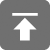# 工学1号馆

home

## size_t 和其他无符号类型的优点

Wu Yudong    August 27, 2016     C   659

for (T i = 0; i < array_size; ++i) {
A[i] = something_nice;
}


T array_size = something;


• 全部有符号，结果有符号
• 全部无符号，结果无符号
• 混合，结果无符号

(也许在一些历史平台上有点不同，但在过去的20年里应该没问题)

sizeof A / sizeof A


for (T i = 0; i < sizeof A / sizeof A; ++i) {
A[i] = something_nice;
}


T就应该是无符号类型

for (T i = start_value; in_bounds(i); i = update(i)) {
A[i] = something_nice;
}


bool in_bounds(T i) {
return i >= 0 && i < some_value;
}


bool in_bounds(T i) {
return i < some_value;
}


for (size_t i = 41; i < sizeof A / sizeof A; --i) {
A[i] = something_nice;
}